# PVT：可用于密集任务backbone的金字塔视觉transformer！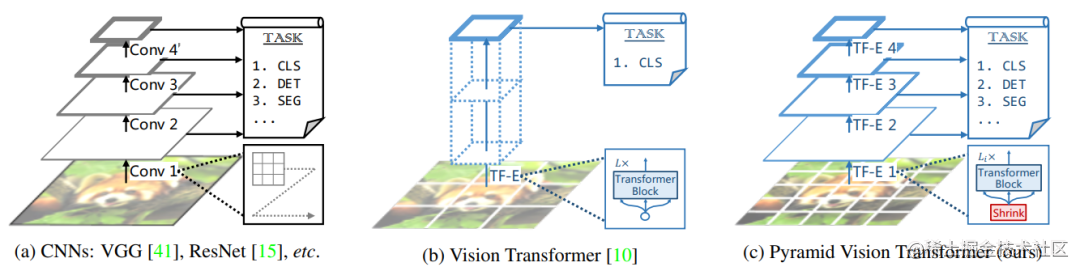CNN结构常用的是一种金字塔架构，如上图所示，CNN网络一般可以划分为不同的stage，在每个stage开始时，特征图的长和宽均减半，而特征维度（channel）扩宽2倍。这主要有两个方面的考虑，一是采用stride=2的卷积或者池化层对特征降维可以增大感受野，另外也可以减少计算量，但同时空间上的损失用channel维度的增加来弥补。但是ViT本身就是全局感受野，所以ViT就比较简单直接了，直接将输入图像tokens化后就不断堆积相同的transformer encoders，这应用在图像分类上是没有太大的问题。但是如果应用在密集任务上，会遇到问题：一是分割和检测往往需要较大的分辨率输入，当输入图像增大时，ViT的计算量会急剧上升；二是ViT直接采用较大patchs进行token化，如采用16x16大小那么得到的粗粒度特征，对密集任务来说损失较大。这正是PVT想要解决的问题，PVT采用和CNN类似的架构，将网络分成不同的stages，每个stage相比之前的stage特征图的维度是减半的，这意味着tokens数量减少4倍，具体结构如下：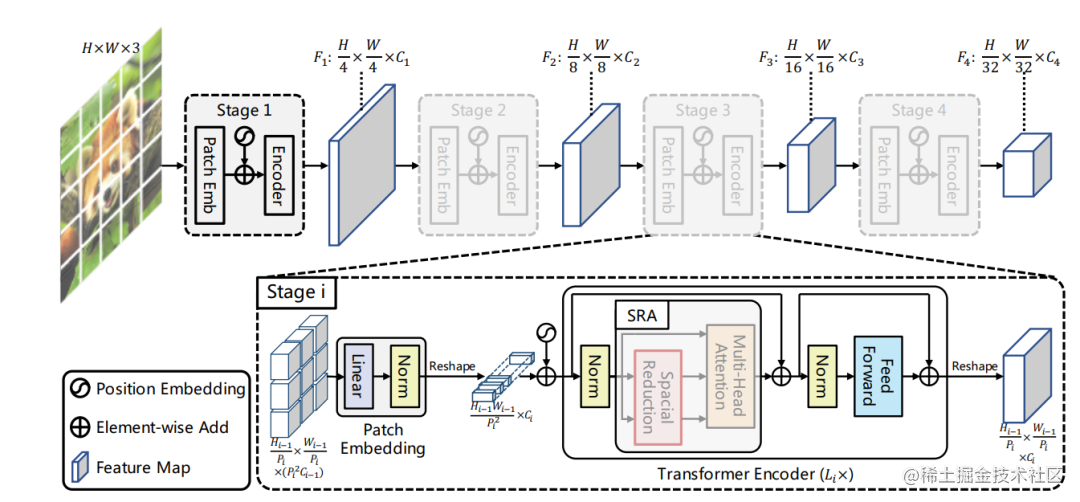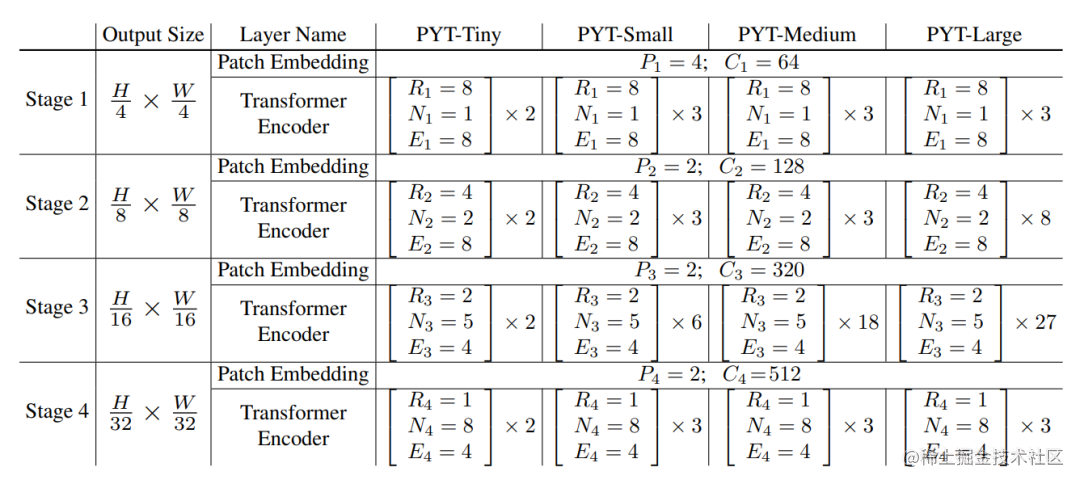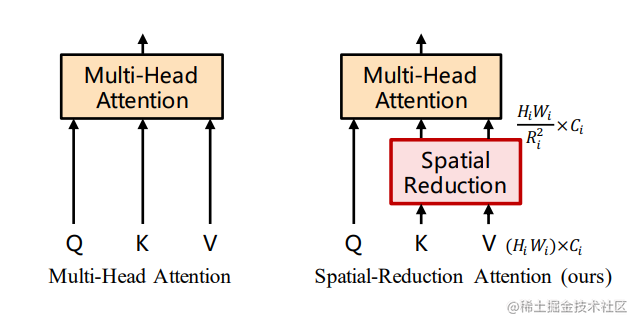``````class Attention(nn.Module):
def __init__(self, dim, num_heads=8, qkv_bias=False, qk_scale=None, attn_drop=0., proj_drop=0., sr_ratio=1):
super().__init__()

self.dim = dim
self.scale = qk_scale or head_dim ** -0.5

self.q = nn.Linear(dim, dim, bias=qkv_bias)
self.kv = nn.Linear(dim, dim * 2, bias=qkv_bias)
self.attn_drop = nn.Dropout(attn_drop)
self.proj = nn.Linear(dim, dim)
self.proj_drop = nn.Dropout(proj_drop)

self.sr_ratio = sr_ratio
# 实现上这里等价于一个卷积层
if sr_ratio > 1:
self.sr = nn.Conv2d(dim, dim, kernel_size=sr_ratio, stride=sr_ratio)
self.norm = nn.LayerNorm(dim)

def forward(self, x, H, W):
B, N, C = x.shape

if self.sr_ratio > 1:
x_ = x.permute(0, 2, 1).reshape(B, C, H, W)
x_ = self.sr(x_).reshape(B, C, -1).permute(0, 2, 1) # 这里x_.shape = (B, N/R^2, C)
x_ = self.norm(x_)
kv = self.kv(x_).reshape(B, -1, 2, self.num_heads, C // self.num_heads).permute(2, 0, 3, 1, 4)
else:
kv = self.kv(x).reshape(B, -1, 2, self.num_heads, C // self.num_heads).permute(2, 0, 3, 1, 4)
k, v = kv, kv

attn = (q @ k.transpose(-2, -1)) * self.scale
attn = attn.softmax(dim=-1)
attn = self.attn_drop(attn)

x = (attn @ v).transpose(1, 2).reshape(B, N, C)
x = self.proj(x)
x = self.proj_drop(x)

return x

PVT具体到图像分类任务上，和ViT一样也通过引入一个class token来实现最后的分类，不过PVT是在最后的一个stage才引入：

``````    def forward_features(self, x):
B = x.shape

# stage 1
x, (H, W) = self.patch_embed1(x)
x = x + self.pos_embed1
x = self.pos_drop1(x)
for blk in self.block1:
x = blk(x, H, W)
x = x.reshape(B, H, W, -1).permute(0, 3, 1, 2).contiguous()

# stage 2
x, (H, W) = self.patch_embed2(x)
x = x + self.pos_embed2
x = self.pos_drop2(x)
for blk in self.block2:
x = blk(x, H, W)
x = x.reshape(B, H, W, -1).permute(0, 3, 1, 2).contiguous()

# stage 3
x, (H, W) = self.patch_embed3(x)
x = x + self.pos_embed3
x = self.pos_drop3(x)
for blk in self.block3:
x = blk(x, H, W)
x = x.reshape(B, H, W, -1).permute(0, 3, 1, 2).contiguous()

# stage 4
x, (H, W) = self.patch_embed4(x)
cls_tokens = self.cls_token.expand(B, -1, -1) # 引入class token
x = torch.cat((cls_tokens, x), dim=1)
x = x + self.pos_embed4
x = self.pos_drop4(x)
for blk in self.block4:
x = blk(x, H, W)

x = self.norm(x)

return x[:, 0]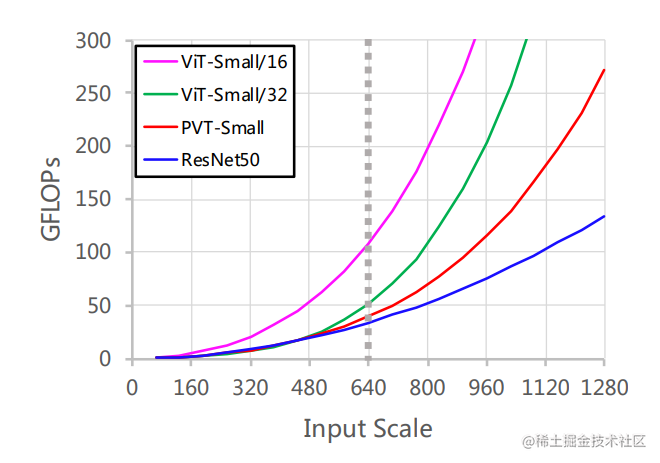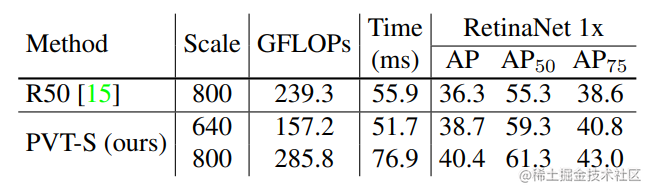## 参考

1. Pyramid Vision Transformer: A Versatile Backbone for Dense Prediction without Convolutions

2. whai362/PVT

3. 大白话Pyramid Vision Transformer

4. You Only Look One-level Feature

5. Swin Transformer: Hierarchical Vision Transformer using Shifted Windows

6. Vision Transformers for Dense Prediction

"未来"的经典之作ViT：transformer is all you need！

Transformer为何能闯入CV界秒杀CNN？

SWA：让你的目标检测模型无痛涨点1% AP

centerX: 用新的视角的方式打开CenterNet

mmdetection最小复刻版(十一)：概率Anchor分配机制PAA深入分析

MMDetection新版本V2.7发布，支持DETR，还有YOLOV4在路上!

CNN：我不是你想的那样

TF Object Detection 终于支持TF2了！

mmdetection最小复刻版(七)：anchor-base和anchor-free差异分析

mmdetection最小复刻版(四)：独家yolo转化内幕